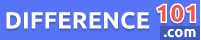Vector Quantity

A vector quantity is a physical quantity that encompasses both magnitude and direction. This means that any vector quantity cannot be explained by a single numerical value alone as it requires both the magnitude and direction of the quantity. For instance, velocity, momentum, and force are all examples of vector quantities in physics. While scalar quantities are represented only by their magnitude or numerical value, vector quantities must be depicted by both their magnitude and direction in order to be fully understood. Vector quantities play a crucial role in physics as they provide a useful framework for explaining and analyzing a range of physical phenomena. Thus, an understanding of vector quantities is essential for anyone pursuing a career or interest in physics.